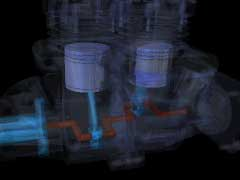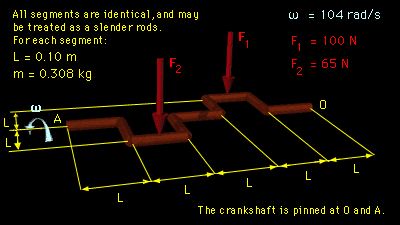Ch 9. 3-D Motion of a Rigid Body Multimedia Engineering Dynamics Translating Coordinates Rotating Coordinates Inertia & Ang. Momentum Equationsof Motion
 Chapter - Particle - 1. General Motion 2. Force & Accel. 3. Energy 4. Momentum - Rigid Body - 5. General Motion 6. Force & Accel. 7. Energy 8. Momentum 9. 3-D Motion 10. Vibrations Appendix Basic Math Units Basic Equations Sections Search eBooks Dynamics Fluids Math Mechanics Statics Thermodynamics Author(s): Kurt Gramoll ©Kurt GramollDYNAMICS - CASE STUDY IntroductionProblem Graphic A two-cylinder piston engine is idling at 1000 rpm when the throttle is opened. How will the asymmetric shape of the crankshaft and the forces exerted on it affect the motion of the crankshaft? What is known: The crankshaft is composed of nine identical segments of steel rod as shown. At the instant the throttle is opened, the crankshaft is in the horizontal plane and is rotating at 104 rad/s. Piston 1, which is firing, exerts a force F1 perpendicular to the horizontal plane. Piston 2, which is compressing fuel for the next cycle, exerts a resisting force F2 in the same direction as F1.Problem Diagram Questions At the instant the throttle is opened, what is the angular acceleration, αz, of the crankshaft? What are the dynamic reaction forces AX and AY from the bearing at point A? Approach Use the equations of rotational motion in 3-D to develop three scalar equations summing the moments about a coordinate system fixed at O. Solve the three equations simultaneously for the three unknowns: αz, AX, AY. Assume each section of the crankshaft is a slender rod.

Practice Homework and Test problems now available in the 'Eng Dynamics' mobile app
Includes over 400 problems with complete detailed solutions.
Available now at the Google Play Store and Apple App Store.